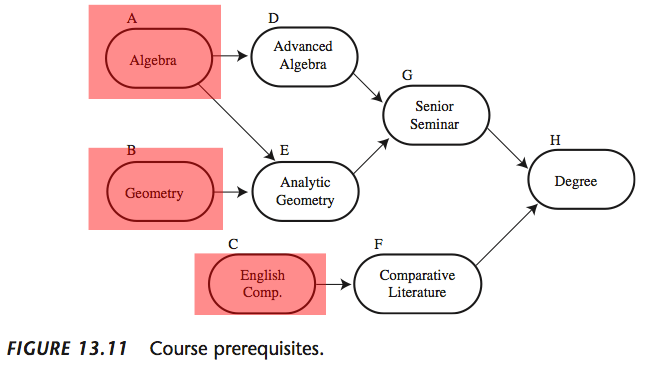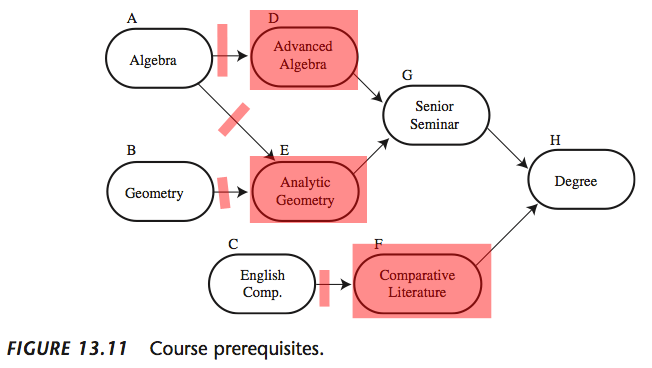## The Problem

Given a set of events, each of which having 0 or more prerequisite events, output a suggested ordering such that all of a given events pre-requisites appear before it.

## Data Representation

• Not super intuitive, but a directed graph works very well for this problem
• Directed meaning that edges are not bidirectional
• Each event represents a node in the graph
• An edge going from event A to event B denotes that A is a pre-requisite of B

## Building the graph

• We can use a graph structure similar to that in week 2, with a few minor modifications (seen later)
• A full description of this structure can be found here
• Simply read in the end points and connect them on the graph

## Algorithm for generating sequence

• There is a well known algorithm for sorting graph nodes based on pre-requisites
• Known as topological sort
• Produces a linear ordering of vertices such that for every directed edge uv from vertex u to vertex v, u comes before v in the ordering
• Note that this is only possible if the given graph is a DAG (directed acyclic graph)
• Also note that more than 1 valid topological sort may exist for a given DAG

## The Algorithm

• The first step is to find all events with no pre-requisites, these will always come first in the ordering
•## The Algorithm

• We add these events to a queue to be processed, and begin a loop while the queue is not empty
• For each iteration, we pop an element off the queue, and disconnect it from its children
• The reason for this is because it has already been processed, so we are “fulfilling” the pre-requisite

## The Algorithm## The Algorithm

• We then check if this disconnecting has caused any nodes to have fulfilled pre-requisites
•## The Algorithm

• Continue this way until we have processed every node in the graph
• At this point we have our ordering
• Our base graph class just needs a way of checking how many incoming edges each node has and we can implement this algorithm